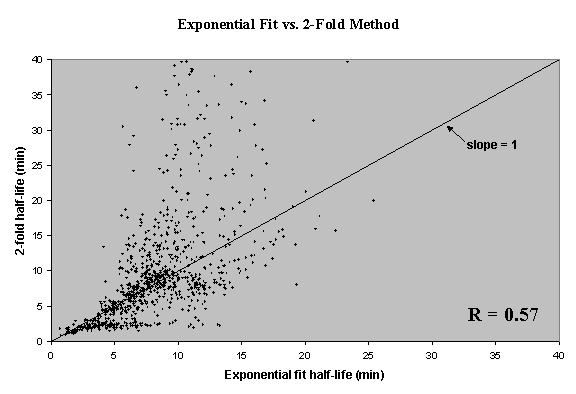Half-life calculation by non-linear least squares curvefitting

In order to improve the statistical rigor and accuracy of the half-life calculation, the data for each RNA was fitted to an exponential function (of the form A = A0ekt) using a non-linear least squares algorithm implented in MATLAB (function nlinfit in the statistics toolbox which uses the Gauss-Newton method).  The function nlparci was then used to estimate 95% confidence intervals for the two parameters (A0,low, klow, A0,hi, and khi) which were then used to calculate upper and lower bounds of the half lives.  Half-lives were calculated for 2,679 RNAs for which A0,low was positive (95% confidence of detection) and khi was negative (95% confidence of decrease).  Half-lives are reported in minutes.  907 half-lives calculated by both methods are plotted in figure 1.

Transcripts which fall along the line fit an exponential degradation pattern, whereas those that fall off the line do not. Those above the line degrade more slowly at the beginning of the timecourse (over their first 2-fold change) and more quickly later on. Those below the line have the opposite pattern.